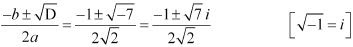# Class 11 Maths NCERT Solutions for Chapter 5 Complex Numbers and Quadratic Equations Exercise 5.3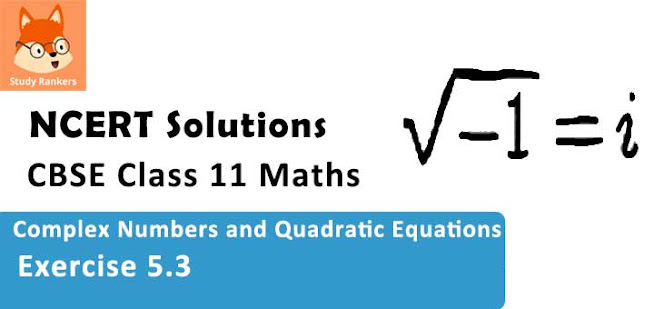### Complex Numbers and Quadratic Equations Exercise 5.3 Solutions

1. Solve the equation x2 + 3 = 0

Solution

The given quadratic equation is x2 + 3 = 0
On comparing the given equation with ax2 + bx + c = 0, we obtain
a = 1, b = 0, and c = 3
Therefore, the discriminant of the given equation is
D = b2 – 4ac = 02 – 4 × 1 × 3 = –12
Therefore, the required solutions are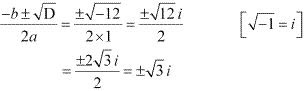2. Solve the equation 2x2 + x + 1 = 0

Solution

The given quadratic equation is 2x2 + x + 1 = 0
On comparing the given equation with ax2 + bx + c = 0, we obtain
a = 2, b = 1, and c = 1
Therefore, the discriminant of the given equation is
D = b2 – 4ac = 12 – 4 × 2 × 1 = 1 – 8 = –7
Therefore, the required solutions are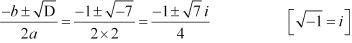3. Solve the equation x2 + 3x + 9 = 0

Solution

The given quadratic equation is x2 + 3x + 9 = 0
On comparing the given equation with ax2 + bx + c = 0, we obtain
a = 1, b = 3, and c = 9
Therefore, the discriminant of the given equation is
D = b2 – 4ac = 32 – 4 × 1 × 9 = 9 – 36 = –27
Therefore, the required solutions are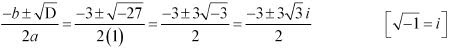4. Solve the equation –x2 + x – 2 = 0

Solution

The given quadratic equation is –x2 + – 2 = 0
On comparing the given equation with ax2 + bx + c = 0, we obtain
a = –1, b = 1, and c = –2
Therefore, the discriminant of the given equation is
D = b2 – 4ac = 12 – 4 × (–1) × (–2) = 1 – 8 = –7
Therefore, the required solutions are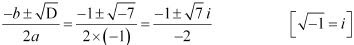5. Solve the equation x2 + 3x + 5 = 0

Solution

The given quadratic equation is x2 + 3x + 5 = 0
On comparing the given equation with ax2 + bx + c = 0, we obtain
a = 1, b = 3, and c = 5
Therefore, the discriminant of the given equation is
D = b2 – 4ac = 32 – 4 × 1 × 5 =9 – 20 = –11
Therefore, the required solutions are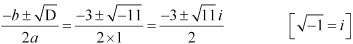6. Solve the equation x2 – x + 2 = 0

Solution

The given quadratic equation is x2 – x + 2 = 0
On comparing the given equation with ax2 + bx + c = 0, we obtain
a = 1, b = –1, and c = 2
Therefore, the discriminant of the given equation is
D = b2 – 4ac = (–1)2 – 4 × 1 × 2 = 1 – 8 = –7
Therefore, the required solutions are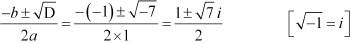7. Solve the equation √2x2 + x + √2 = 0

Solution

The given quadratic equation is √2x2 + x + √2 = 0
On comparing the given equation with ax2 + bx + c = 0, we obtain
a = √2, b = 1, and c = √2
Therefore, the discriminant of the given equation is
D = b2 - 4ac = 12 - 4 × √2 × √2 = 1 - 8 =-7
Therefore, the required solution are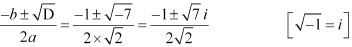8. Solve the equation √3x2 - √2x + 3√3 = 0

Solution

The given quadratic equation is √3x2 - √2x + 3√3 = 0
On comparing the given equation with ax2 + bx + c = 0, we obtain
a = √3, b = -√2, and c = 3√3
Therefore, the discriminant of the given equation is
d = b2 - 4ac = (-√2)2 - 4(√3)(3√3) = 2 - 36 = -34
Therefore, the required solution are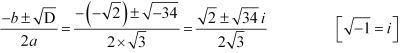9. Solve the equation x2 + x + 1/√2 = 0

Solution

The given quadratic equation is x2 + x+ 1/√2 = 0
This equation can also be written as √2x2 + √2x+ 1 = 0
On comparing this equation  with ax2 + bx + c = 0, we obtain
a = √2, b = √2, and c = 1
∴ Discriminant (D) = b2 - 4ac = (√2)2 - 4×√2 ×1 = 2 - 4√2
Therefore, the required solutions are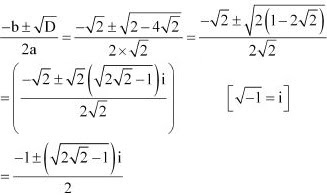10. Solve the equation x2 + x/√2 + 1 = 0

Solution

The given quadratic equation is x2 + x/√2 + 1 = 0
This equation can also be written as √2x2 + x + √2 = 0
On comparing this equation with ax2 +bx + c = 0, we obtain
a = √2, b = 1, and c = √2
∴ Discriminant (D) = b2 - 4ac = 12 - 4×√2×√2 = 1 - 8 = -7
Therefore, the required solutions are